Question

LEAD IS A SOFT, DENSE METAL WITH A SPECIFIC HEAT OF 0.028KCAL/KGC, A MELTING POINT OF 328.0C, AND A HEAT FUSION OF5.5KCAL/KG

LEAD IS A SOFT, DENSE METAL WITH A SPECIFIC HEAT OF 0.028KCAL/KGC, A MELTING POINT OF 328.0C, AND A HEAT FUSION OF5.5KCAL/KG. HOW MUCH HEAT MUST BE PROVIDED TO MELT A 250.0KG SAMPLE OF LEAD WITH A TEMPERATURE OF 20.0C?

Figure the heat to heat if from 20C to the melting point (Q=mc deltaT)
Add the heat needed to melt it at the melting point (Q=heatfusion*mass)

I CAME UP WITH 1,640KCAL I AM RIGHT?

No. Break it down in to calculations as I laid out, and let me see them.

We need at least 10 more requests to produce the answer.

0 / 10 have requested this problem solution

The more requests, the faster the answer.

All students who have requested the answer will be notified once they are available.

Earn Coins

Coins can be redeemed for fabulous gifts.

Similar Homework Help Questions
• lead is a soft, dense metal with a specific heat of 0.028 kcal/kgC, a melting point of 328.0C,...

lead is a soft, dense metal with a specific heat of 0.028 kcal/kgC, a melting point of 328.0C, and a heat fusion of 5.5 kcal/kg. how much heat must be provided tomelt a 250.0 kg sample of lead with a temperature of 20.0 ?please show work

• Lead is a soft, dense metal with a specific heat of 0.028 kcal/kg·C°, a melting point of 328.0°C, and a heat of fusion of 5.5 kcal/kg

Lead is a soft, dense metal with a specific heat of 0.028 kcal/kg·C°, a melting point of 328.0°C, and a heat of fusion of 5.5 kcal/kg. How much heat must be provided to melt a 202.2 kg sample of lead with a temperature of 20.0°C?

• lead has specific heat of

lead has specific heat of .028kcal/kgC, melting point of 328 degrees C and a heat fusion of 5.5 kcal/kg. how much heat must be provided to melt a 250kg sample of lead with a temperature of 20 degrees Celsius?

• The specific heat capacity of solid lead is 0.159J/g°C, the molar enthalpy of fusion is 5.0kJ/mol, and the melting point of lead is 328°C

The specific heat capacity of solid lead is 0.159J/g°C, the molar enthalpy of fusion is 5.0kJ/mol, and the melting point of lead is 328°C. Calculate the total energy required to change 100g of lead at 25°C to molten lead at it's melting point. I will be happy to critique your work or thinking. See the other posts in this series for hints. Fair enough, the least I can do is try it. Basically I will find the heat required to...

• 2 The specific heat of mercury is 138 J/kgC. The following is calorimeter data: 1.00 kg...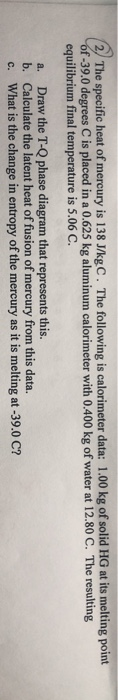2 The specific heat of mercury is 138 J/kgC. The following is calorimeter data: 1.00 kg of solid HG at its melting point f-39.0 degrees C is placed in a 0.625 kg aluminum calorimeter with 0.400 kg of water at 12.80 C. The resulting equilibrium final temperature is 5.06 C. a. Draw the T-Q phase diagram that represents this b. Calculate the latent heat of fusion of mercury from this data. c. What is the change in entropy of the...

• Use the following table of thermal physics specs. Latent specific melting/freezing heat of substance heat point...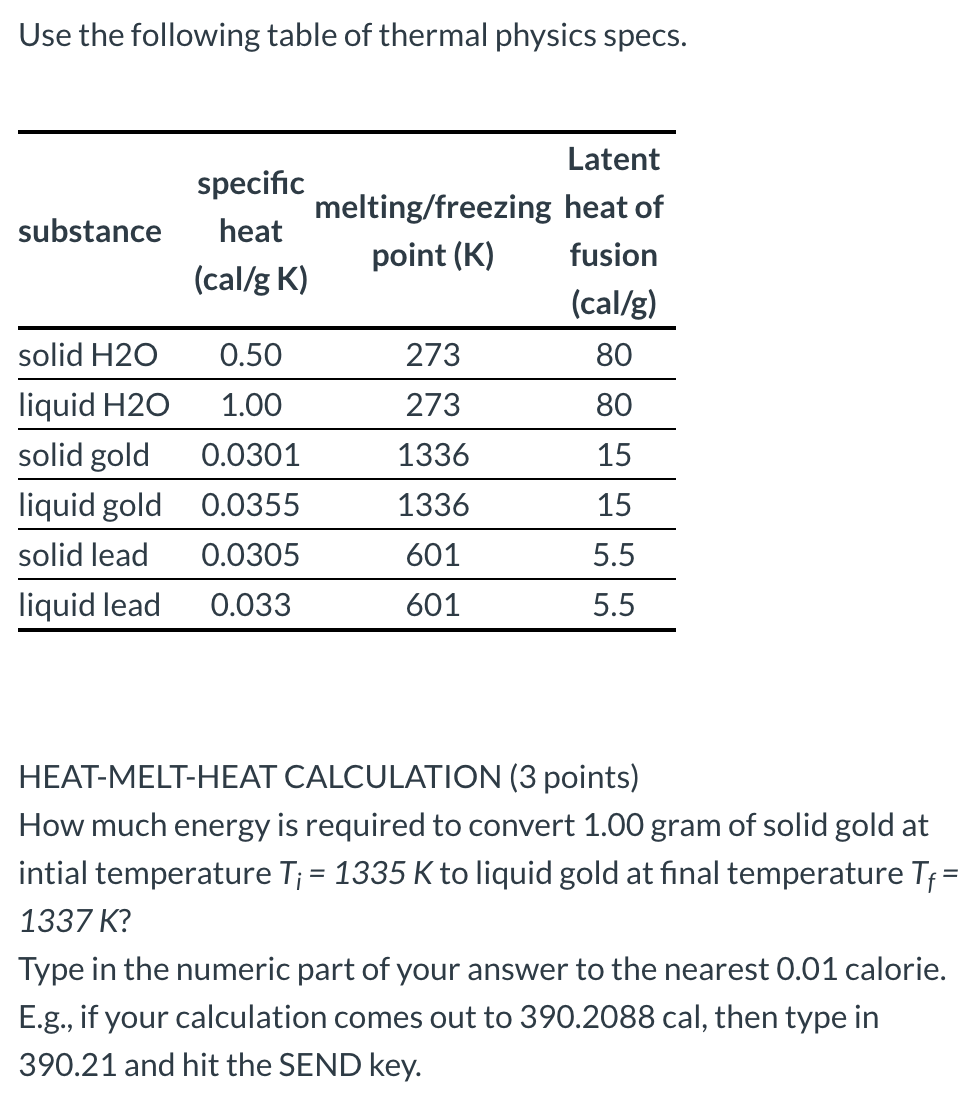Use the following table of thermal physics specs. Latent specific melting/freezing heat of substance heat point (K) fusion (cal/g K) (cal/g) solid H2O 0.50 273 80 liquid H2O 1.00 273 80 solid gold 0.0301 1336 15 liquid gold 0.0355 1336 15 solid lead 0.0305 601 5.5 liquid lead 0.033 601 5.5 HEAT-MELT-HEAT CALCULATION (3 points) How much energy is required to convert 1.00 gram of solid gold at intial temperature T; = 1335 K to liquid gold at final temperature...

• 63. Use the following table of thermal physics specs. substance specific heat melting/freezing Latent heat of...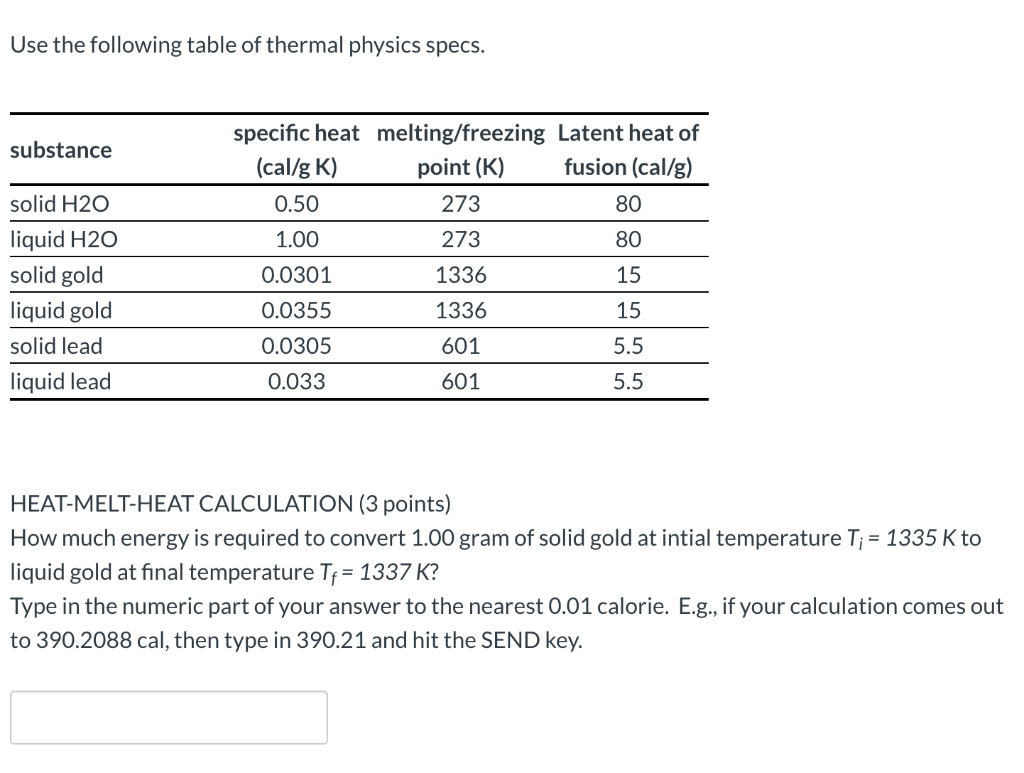63. Use the following table of thermal physics specs. substance specific heat melting/freezing Latent heat of (cal/g K) point (K) fusion (cal/g) 0.50 273 80 1.00 273 80 0.0301 1336 15 solid H2O liquid H20 solid gold liquid gold solid lead liquid lead 0.0355 1336 15 0.0305 601 5.5 0.033 601 5.5 HEAT-MELT-HEAT CALCULATION (3 points) How much energy is required to convert 1.00 gram of solid gold at intial temperature T; = 1335 K to liquid gold at final...

• Chemistry 1516R Problem Set QUESTIONS For water, the molar heat of fusion is 6.01 kJ/mol and...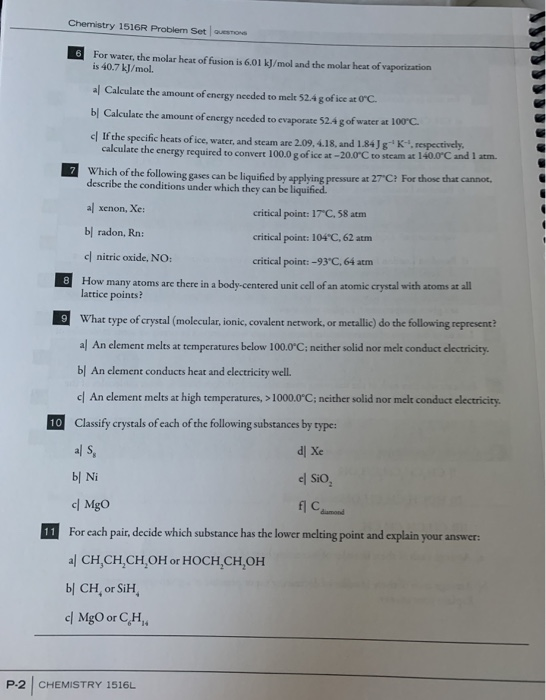Chemistry 1516R Problem Set QUESTIONS For water, the molar heat of fusion is 6.01 kJ/mol and the molar heat of vaporization is 40.7 kJ/mol. al Calculate the amount of energy needed to melt 52.4g of ice at O'C b Calculate the amount of energy needed to evaporate 52.4 g of water at 100°C c If the specific heats of ice, water, and steam are 2.09.4.18 and 1.84J ' respectively, calculate the energy required to convert 100.0 g of iscat -...

• ak home Scraping ice off the window of my car is no fun, how much heat...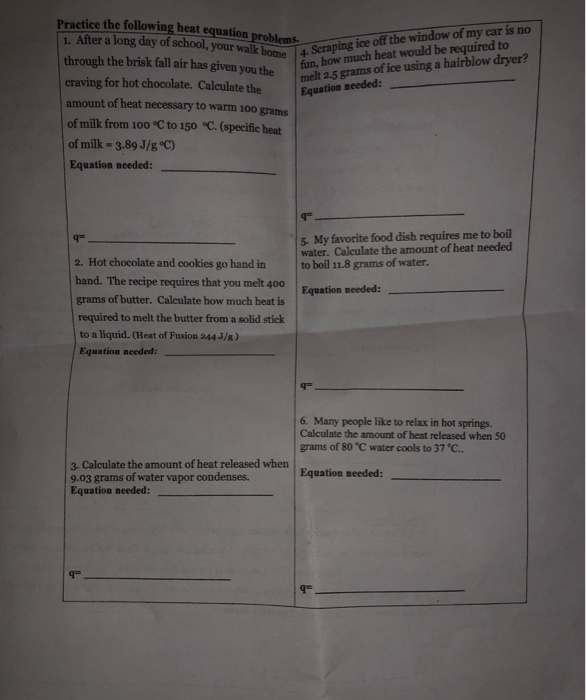ak home Scraping ice off the window of my car is no fun, how much heat would be required to melt 2-5 grams of ice using a hairblow dryer? Practice the following heat equation problems. 1. After a long day of school, your walk home 4. Scrap through the brisk fall air has given you the craving for hot chocolate. Calculate the Equation nece amount of heat necessary to warm 100 grams of milk from 100°C to 150 °C. (specific...

• While reading the story, consider the culture (or sub culture) and related communication styles the story...While reading the story, consider the culture (or sub culture) and related communication styles the story reveals. Consider too, possibly, the values, behavioral norms, social practices, social artifacts, etc. After reading the story through the lens of this idea, please compose a full academic length (evidence-based 7 to 11 sentence long) paragraph which addresses the following prompt: What does the story reveal about the culture it portrays and/OR the communication styles the culture shares? In other words, what does the...